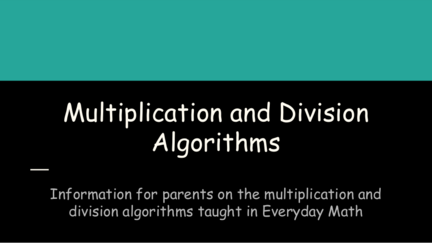# Multiplication and Division AlgorithmsContributed by:This includes Algorithms that generally involve repeating a series of steps over and over, as in the borrowing and carrying algorithms and in the long multiplication and division.
1. Multiplication and Division
Algorithms
Information for parents on the multiplication and
division algorithms taught in Everyday Math
2. What is an algorithm?
An algorithm is a set of rules for solving a math problem
which, if done properly, will give a correct answer each
time. Algorithms generally involve repeating a series of
steps over and over, as in the borrowing and carrying
algorithms and in the long multiplication and division
(Information from: instruction.aaps.k12.mi.us/EM_parent_hdbk/algorithms.html)
3. Why do we teach multiple algorithms?
Researchers working in the 1970s and 1980s showed that U.S. children often learn standard
computational algorithms with very little understanding (Brown & Burton, 1978; Van Lehn 1983,
1986). Other researchers found that the traditional approach to teaching computation engenders
beliefs about mathematics that impede further learning (Hiebert, 1984; Cobb, 1985; Baroody &
Ginsburg, 1986).
On the other hand, Kamii and others demonstrated that students are capable of inventing their own
effective and meaningful methods for computation (Kamii, 1985; Madell, 1985; Kamii & Joseph,
1988; Cobb & Merkel, 1989; Resnick, Lesgold, & Bill, 1990; Carpenter, Fennema, & Franke, 1992).
Furthermore, these experiences were found to improve understanding of place value and enhance
estimation and mental computation skills.
● Most familiar algorithm to adults
● Multiplies from right-to-left
● Regroup as needed
● Below is a video demonstrating the traditional algorithm for multiplication:
https://vlc.uchicago.edu/resources/9697
5. Multiplication - Partial Products Algorithm
● Based on the distributive property of multiplication
● Multiply each digit of one factor with each digit of the other factor
● Below is a video demonstrating the Partial Products Algorithm: http://everydaymath.
uchicago.edu/teaching-topics/computation/mult-part-prod/
6. Lattice Multiplication
● This method has been used for
hundreds of years
● This method is an alternative method
for long multiplication
● Using a grid, or lattice, you are able to
multiply large numbers easily
● Multiply the top digits by the digits on
the side. Then add along the diagonal
lines.
● Below is a video demonstrating lattice multiplication:
http://everydaymath.uchicago.edu/teaching-
topics/computation/mult-lattice/
● Familiar to adults and children
● Place the dividend inside a division
bracket, and the divisor outside and to
the left of the bracket.
● Make a series of estimates and record
the results. Then subtract the result
from the number above it.
● If there is a remainder, it is written
next to the quotient at the end of the
problem.
● Below is a video demonstrating the Traditional Algorithm for Division:
8. Division - Partial Quotients
● At each step find a partial
have found all of the partial
● The better the estimates, the
fewer the steps it takes to
solve the problem.
● Students who struggle with
basic facts can find correct